# Fraction calculator

The calculator performs basic and advanced operations with fractions, expressions with fractions combined with integers, decimals, and mixed numbers. It also shows detailed step-by-step information about the fraction calculation procedure. Solve problems with two, three, or more fractions and numbers in one expression.

## Result:

### 4 - 23/7 = 11/7 = 1 4/7 ≅ 1.5714286

Spelled result in words is eleven sevenths (or one and four sevenths).

### How do you solve fractions step by step?

1. Conversion a mixed number 2 3/7 to a improper fraction: 2 3/7 = 2 3/7 = 2 · 7 + 3/7 = 14 + 3/7 = 17/7

To find new numerator:
a) Multiply the whole number 2 by the denominator 7. Whole number 2 equally 2 * 7/7 = 14/7
b) Add the answer from previous step 14 to the numerator 3. New numerator is 14 + 3 = 17
c) Write a previous answer (new numerator 17) over the denominator 7.

Two and three sevenths is seventeen sevenths
2. Subtract: 4 - 17/7 = 4/1 - 17/7 = 4 · 7/1 · 7 - 17/7 = 28/7 - 17/7 = 28 - 17/7 = 11/7
For adding, subtracting, and comparing fractions, it is suitable to adjust both fractions to a common (equal, identical) denominator. The common denominator you can calculate as the least common multiple of both denominators - LCM(1, 7) = 7. In practice, it is enough to find the common denominator (not necessarily the lowest) by multiplying the denominators: 1 × 7 = 7. In the next intermediate step, the fraction result cannot be further simplified by canceling.
In words - four minus seventeen sevenths = eleven sevenths.

#### Rules for expressions with fractions:

Fractions - use the slash “/” between the numerator and denominator, i.e., for five-hundredths, enter 5/100. If you are using mixed numbers, be sure to leave a single space between the whole and fraction part.
The slash separates the numerator (number above a fraction line) and denominator (number below).

Mixed numerals (mixed fractions or mixed numbers) write as non-zero integer separated by one space and fraction i.e., 1 2/3 (having the same sign). An example of a negative mixed fraction: -5 1/2.
Because slash is both signs for fraction line and division, we recommended use colon (:) as the operator of division fractions i.e., 1/2 : 3.

Decimals (decimal numbers) enter with a decimal point . and they are automatically converted to fractions - i.e. 1.45.

The colon : and slash / is the symbol of division. Can be used to divide mixed numbers 1 2/3 : 4 3/8 or can be used for write complex fractions i.e. 1/2 : 1/3.
An asterisk * or × is the symbol for multiplication.
Plus + is addition, minus sign - is subtraction and ()[] is mathematical parentheses.
The exponentiation/power symbol is ^ - for example: (7/8-4/5)^2 = (7/8-4/5)2

#### Examples:

subtracting fractions: 2/3 - 1/2
multiplying fractions: 7/8 * 3/9
dividing Fractions: 1/2 : 3/4
exponentiation of fraction: 3/5^3
fractional exponents: 16 ^ 1/2
adding fractions and mixed numbers: 8/5 + 6 2/7
dividing integer and fraction: 5 ÷ 1/2
complex fractions: 5/8 : 2 2/3
decimal to fraction: 0.625
Fraction to Decimal: 1/4
Fraction to Percent: 1/8 %
comparing fractions: 1/4 2/3
multiplying a fraction by a whole number: 6 * 3/4
square root of a fraction: sqrt(1/16)
reducing or simplifying the fraction (simplification) - dividing the numerator and denominator of a fraction by the same non-zero number - equivalent fraction: 4/22
expression with brackets: 1/3 * (1/2 - 3 3/8)
compound fraction: 3/4 of 5/7
fractions multiple: 2/3 of 3/5
divide to find the quotient: 3/5 ÷ 2/3

The calculator follows well-known rules for order of operations. The most common mnemonics for remembering this order of operations are:
PEMDAS - Parentheses, Exponents, Multiplication, Division, Addition, Subtraction.
BEDMAS - Brackets, Exponents, Division, Multiplication, Addition, Subtraction
BODMAS - Brackets, Of or Order, Division, Multiplication, Addition, Subtraction.
GEMDAS - Grouping Symbols - brackets (){}, Exponents, Multiplication, Division, Addition, Subtraction.
Be careful, always do multiplication and division before addition and subtraction. Some operators (+ and -) and (* and /) has the same priority and then must evaluate from left to right.

## Fractions in word problems:

• Square metal sheetWe cut out four squares of 300 mm side from a square sheet metal plate with a side of 0,7 m. Express the fraction and the percentage of waste from the square metal sheet.
• In bucket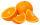In bucket of 87 oranges 1/3 are spoiled ones and the remaining are eaten by the member of a family. How many oranges are eaten by the member of family?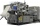Erika’s career consists of filing, typing and answering phones. She spends 1/6 of her time filing and 5/8 of her time typing. What fraction of her time does she spend answering phone calls?
• Sugar 8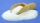Heather has 2 cups of powdered sugar. She sprinkles 3/5 of the sugar onto a plate of brownies and sprinkles the rest into a plate of lemon cookies. How much sugar does Heather sprinkle on the brownies? How much sugar does Heather sprinkle on the lemon coo
• Claire and bookAfter Claire has read the first 5/8 of the book, there are 120 pages left to read. What is the total number of pages of the book?
• CurtainMrs. Lazo bought 9 1/8 m curtain cloth. She used 3 5/6 m to make a curtain for their bedroom. How many meters of cloth were not used?
• Half of 2Half of Ethan’s string is equal to 2/3 of Kayla’s string. The total length of their strings is 10 feet. How much longer is Ethan’s string than Kayla’s?
• Fractions and mixed numerals(a) Convert the following mixed numbers to improper fractions. i. 3 5/8 ii. 7 7/6 (b) Convert the following improper fraction to mixed number. i. 13/4 ii. 78/5 (c) Simplify these fractions to their lowest terms. i. 36/42 ii. 27/45 2. evaluate following ex
• ClarissaClarissa read 1/2 of a book in the morning, and she read some more at night. By the end of the day, she still had 1/3 of the book left to read. How much of the book did Clarissa read at night?
• Honey 2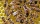The baker has 3/4 liter of honey and decided to bake a few honeycombs and biscuits from it. 1/6 liter of honey was needed for honeycombs and 1/4 liter for biscuits. How much honey did she have left after baking honeycombs and biscuits?
• Evaluate fractionsThe difference  of 5 1/2 and 2/3 is added to the product of 5/6 and 1/2
• Farmer 5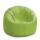Farmer Joe ordered 3 bags of soil last month. Each bag weighed 4 ⅖ kilograms. He used the first bag in a week. At the end of this month, there were 2 ¾ kilograms of soil left in the second bag and ⅞ kilograms of soil left in the third bag. How much soil w
• SchoolThere are 150 pupils in grade 5 . 2/3 of them are female. By what fractions are the males?